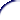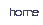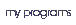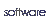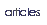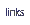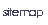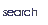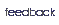## Programming in User RPL - First concepts

Now that you've payed 270 bucks for you HP48GX, it should at least be able to do everything you need. Several funcitions are already avaliable, but probably you'll need to do something the HP cannot do automatically. Say for example, you have to calculate the determinant of a symbolic matrix. Since the HP only supports numerical matrices, you'll have to calculate it manually. If you need to do that only once, OK, but what to do if you need several calculations, always doing the same thing, pressing the same keys, but with different inputs?

That's why the HP48 is different from other calculators. It is programmable. It means it can learn how to do something. In the example above, you could write a program that calculates the determinat of a symbolic matrix when you give the elements. So, to calculate the determinat, you would just need to enter the elements and run the program. Want to calculate with different elements? Just key in them and run the program again. You'll get the new results automatically.

Basically, programmable calculator can save you time.

On the HP48, programs are delimited by `<< >>` (to put `<< >>`, press left-shift-Minus.) Inside, there is a sequence of objects, separeted by spaces. Depending on the object type, some action is taken, according to the table:

 Object Action Command Run Number Put into stack Algebraic Put into stack String Put into stack List Put into stack Program Put into stack Global name (between `''`) Put into stack Global name (without `''`) Put into stack and automatically evaluated Local Name (between `''`) Put into stack Local name (without `''`) Contents put into stack

Let's see a simple example of a program. Suppose you have a 52 gallon cylindrical water heater and you wish to determine how much energy is being lost because of poor insulation. For that, you can use the formula

q = hAT

where q is the heat loss from the heater (btu per hour); h is the heat-transfer coeficient, in our case 0.47; A is the total surface area of the cylinder, in our example 30; and T is the temperature difference.

The values of h and A will remain constant in or case, but we want to calculate the heat loss for various temperature differences. You could key in the values every time and multiply them, but this task can be simplified with programming. Let's create a simple program to calcule the heat loss for the heater when the temperature difference is on the stack. Key in the following program, and press `ENTER`:

`<< 30 * .47 * >>`

Lets examine this program carefully. The first thing is the number 30. Since it's a number, it will be put into the stack. Now the stack contains the temperature difference (which you entered) and 30, the surface area, that the program entered. The next object is a command, `*`. It will multiply the two numbers on the stack, calculating A X T. Following, there is another number, which will be put into the stack. And finnaly, another command, again `*`. Now the program has multiplied A X T by h, getting the result q.

Let's see if this works. If you have not already entered the program, enter it now. You should see the program on level 1 of the stack. To save it as a variable, type `'HT'`, press `ENTER` then `STO`. Now press `VAR`. See `HT` on the first menu key? Now, enter 15 and press the first softkey. Did you get 211.5? Now, if you wanted to calculate again for a temperature difference of 18 degrees, just enter 18 and press the menu key. You should get 253.8.

To see how easy it would be if you had to calculate several times, calculate the heat loss for the differences of temperature of 10, 12, 14, 16, 18 and 20. The answers are 141, 169.2, 197.4, 225.6, 253.8 and 282.

### Stack Manipulation

Of the several commands that can be included in programs, some of the most important ones are the ones that manipulate the stack, ie, change the order of the elements, removes some elements or make copies of some of them. The HP provides 13 commands for manipulating the stack. It is not recommend that you know what them do. It is essential to know what them do. Was I clear? You must know how to use them and their effect. Anyway, it isn't so difficult. Here they are:

 Command Effect Example Before After `DUP` Makes a copy of the object on level 1 of the stack `2: 1: a` `2: a1: a` `DUP2` Makes copies of the objects on levels 1 and 2 of the stack `4: 3: 2: a1: b` `4: a3: b2: a1: b` `DUPN` Makes copies of n objects on the stack, starting at level 2 (n is on level 1) ```6: 5: 4: a3: b2: c1: 3``` ```6: a5: b4: c3: a2: b1: c``` `DROP` Drops the object on level 1 of the stack `2: a1: b` `2: 1: a` `DROP2` Drops the objects of levels 1 and 2 of the stack `3: a2: b1: c` `3: 2: 1: a` `DROPN` Drops the n first objects of the stack, starting on level 2 (n is on the stack) `5: a4: b3: c2: d1: 3` `5: 4: 3: 2: 1: a` `OVER` Returns a copy of the object on level 2 `3: 2: a1: b` `3: a2: b1: a` `PICK` Returns a copy of the object on level n+1 of the stack (n is on the stack) 4: a3: b2: c1: 3 4: b3: c2: c1: a `SWAP` Exchanges levels 1 and 2 2: a1: b 2: b1: a `ROLL` Moves object on level n+1 to level 1 `5: a4: b3: c2: d1: 4` `5: 4: b3: c2: d1: a` `ROLLD` Moves object on level 2 to level n+1 ```5: a4: b3: c2: d1: 4``` ```5: 4: d3: a2: b1: c``` `ROT` Moves object on level 3 to level 1 (equivalent to ```3 ROLL```) `3: a2: b1: c` `3: b2: c1: a` `DEPTH` Returns the number of elements on the stack `4: 3: a2: b1: c` ```4: a3: b2: c1: 3```

Now let's see another very simple program. It calculates the hypotenuse of a right triangle, given the tow cates on the stack.

`<< SQ SWAP SQ +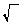>>`

#### Exercises

1. What happens when the following programs are run?
1. ```<< << << 1 2 + >> >> EVAL >>```
2. ```<< << << 1 2 + >> EVAL >> >>```
3. ```<< << << 1 2 + >> EVAL >> EVAL >>```
4. ```<< << << 1 2 + >> >> EVAL EVAL >>```
5. ```<< << 1 << 2 + >> >> EVAL >>```
6. ```<< << 1 << 2 + >> EVAL >> EVAL >>```
2. Design a program that, when given the radius of a sphere in level 1, calculates the volume of it. ()
3. Write a program to convert from ºF to ºC. (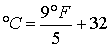)
4. Write a program to convert from ºC to ºF
5. Write a program to calculate the parallel resistance RP of two resistors R1 and R2, whose values are on the stack, using the formula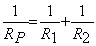6. Design a program that calculates the parallel resistance of two resistors on the stack, using the formula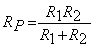. OBS: Yes, both formulas are valid, and it isn't difficult to prove that.
7. Write a program to calculate the determinant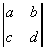, assuming a, b, c and d are on the stack, in this order.
8. Write a program to calculate the distance of two points (x1,y1) and (x2,y2), assuming x1, x2, y1 and y2 are on the stack in this order.
9. Write a program to find one root of a quadratic equation, assuming a, b and c are on the stack.
10. Modifiy the above program so that it finds both roots of the equation.
11. Write a program to find the surface area of a rectangular box, using the equation S = 2(hw + hd + wd)